## Algorithms for the Travelling Salesman ProblemThe Traveling Salesman Problem (TSP) is the challenge of finding the shortest path or shortest route for a salesperson to take, given a starting point, a number of cities (nodes), and optionally an ending point. It is a well-known algorithmic problem in the fields of computer science and operations research, with important real-world applications for logistics and delivery businesses.

There are obviously a lot of different routes to choose from, but finding the best one—the one that will require the least distance or cost—is what mathematicians and computer scientists have spent decades trying to solve.

It’s much more than just an academic problem. Finding more efficient routes using route optimization algorithms increases profitability for delivery businesses, and reduces greenhouse gas emissions because it means less distance traveled.

In theoretical computer science, the TSP has commanded so much attention because it’s so easy to describe yet so difficult to solve. The TSP is known to be a combinatorial optimization problem that’s an NP-hard problem, which means that the number of possible solution sequences grows exponential with the number of cities. Computer scientists have not found any algorithm that can solve this problem in polynomial time, and therefore rely on approximation algorithms to try numerous permutations and select the shortest route with the minimum cost.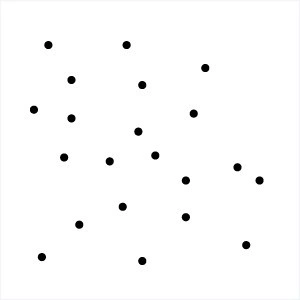The main problem can be solved by calculating every permutation using a brute force approach and selecting the optimal solution. However, as the number of destinations increases, the corresponding number of roundtrips grows exponentially and surpasses the capabilities of even the fastest computers. With 10 destinations, there can be more than 300,000 roundtrip permutations. With 15 destinations, the number of possible routes could exceed 87 billion.

For larger real-world travelling salesman problems, when manual methods such as Google Maps Route Planner or Excel route planner no longer suffice, businesses rely on approximate solutions that are sufficiently optimized by using fast tsp algorithms that rely on heuristics. Finding the exact optimal solution using dynamic programming is usually not practical for large problems.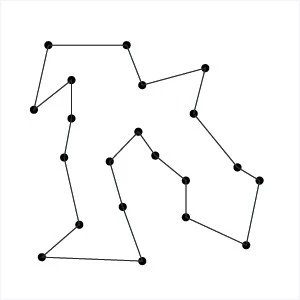## Three popular Travelling Salesman Problem Algorithms

Here are some of the most popular solutions to the Travelling Salesman Problem:

## 1. The brute-force approach

The Brute Force approach, also known as the Naive Approach, calculates and compares all possible permutations of routes or paths to determine the shortest unique solution. To solve the TSP using the Brute-Force approach, you must calculate the total number of routes and then draw and list all the possible routes. Calculate the distance of each route and then choose the shortest one—this is the optimal solution.

This is only feasible for small problems, rarely useful beyond theoretical computer science tutorials.

## 2. The branch and bound method

The branch and bound algorithm starts by creating an initial route, typically from the starting point to the first node in a set of cities. Then, it systematically explores different permutations to extend the route one node at a time. Each time a new node is added, the algorithm calculates the current path's length and compares it to the optimal route found so far. If the current path is already longer than the optimal route, it "bounds" or prunes that branch of the exploration, as it would not lead to a more optimal solution.

This pruning is the key to making the algorithm efficient. By discarding unpromising paths, the search space is narrowed down, and the algorithm can focus on exploring only the most promising paths. The process continues until all possible routes are explored, and the shortest one is identified as the optimal solution to the traveling salesman problem. Branch and bound is an effective greedy approach for tackling NP-hard optimization problems like the travelling salesman problem.

## 3. The nearest neighbor method

To implement the Nearest Neighbor algorithm, we begin at a randomly selected starting point. From there, we find the closest unvisited node and add it to the sequencing. Then, we move to the next node and repeat the process of finding the nearest unvisited node until all nodes are included in the tour. Finally, we return to the starting city to complete the cycle.

While the Nearest Neighbor approach is relatively easy to understand and quick to execute, it rarely finds the optimal solution for the traveling salesperson problem. It can be significantly longer than the optimal route, especially for large and complex instances. Nonetheless, the Nearest Neighbor algorithm serves as a good starting point for tackling the travelling salesman problem and can be useful when a quick and reasonably good solution is needed.

This greedy algorithm can be used effectively as a way to generate an initial feasible solution quickly, to then feed into a more sophisticated local search algorithm, which then tweaks the solution until a given stopping condition.  ‍

## How route optimization algorithms work to solve the Travelling Salesman Problem.

Academics have spent years trying to find the best solution to the Travelling Salesman Problem The following solutions were published in recent years:

• Machine learning speeds up vehicle routing : MIT researchers apply Machine Learning methods to solve large np-complete problems by solving sub-problems.
• Zero Suffix Method : Developed by Indian researchers, this method solves the classical symmetric TSP.
• Biogeography‐based Optimization Algorithm : This method is designed based on the animals’ migration strategy to solve the problem of optimization.
• Meta-Heuristic Multi Restart Iterated Local Search (MRSILS): The proponents of this research asserted that the meta-heuristic MRSILS is more efficient than the Genetic Algorithms when clusters are used.
• Multi-Objective Evolutionary Algorithm : This method is designed for solving multiple TSP based on NSGA-II.
• Multi-Agent System : This system is designed to solve the TSP of N cities with fixed resource.

## Real-world TSP applications

Despite the complexity of solving the Travelling Salesman Problem, it still finds applications in all verticals.

For example, TSP solutions can help the logistics sector improve efficiency in the last mile. Last mile delivery is the final link in a supply chain, when goods move from a transportation hub, like a depot or a warehouse, to the end customer. Last mile delivery is also the leading cost driver in the supply chain. It costs an average of $10.1, but the customer only pays an average of$8.08 because companies absorb some of the cost to stay competitive. So bringing that cost down has a direct effect on business profitability.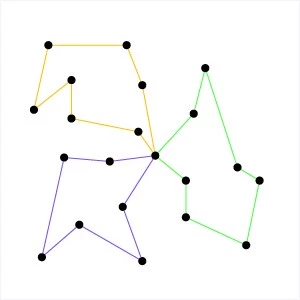Minimizing costs in last mile delivery is essentially in last mile delivery is essentially a Vehicle Routing Problem (VRP). VRP, a generalized version of the travelling salesman problem, is one of the most widely studied problems in mathematical optimization. Instead of one best path, it deals with finding the most efficient set of routes or paths. The problem may involve multiple depots, hundreds of delivery locations, and several vehicles. As with the travelling salesman problem, determining the best solution to VRP is NP-complete.

## Real-life TSP and VRP solvers

While academic solutions to TSP and VRP aim to provide the optimal solution to these NP-hard problems, many of them aren’t practical when solving real world problems, especially when it comes to solving last mile logistical challenges.

That’s because academic solvers strive for perfection and thus take a long time to compute the optimal solutions – hours, days, and sometimes years. If a delivery business needs to plan daily routes, they need a route solution within a matter of minutes. Their business depends on delivery route planning software so they can get their drivers and their goods out the door as soon as possible. Another popular alternative is to use Google maps route planner .

Real-life TSP and VRP solvers use route optimization algorithms that find a near-optimal solutions in a fraction of the time, giving delivery businesses the ability to plan routes quickly and efficiently.

## If you want to know more about real-life TSP and VRP solvers, check out the resources below 👇

Route Optimization API - TSP Solver

Route Optimization API - VRP SolverRelated articles.## Solving the Vehicle Routing Problem (2023)## What Is Route Optimization?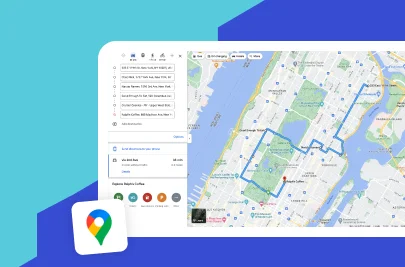## Explained: What is Traveling Salesman Problem (TSP)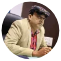• Last Updated: January 24, 2023• A well-known mathematical problem called the Traveling Salesman Problem (TSP) aims to determine the shortest path between a number of places.
• Logistics, transportation, and manufacturing are just a few of the industries where the TSP is useful.
• The number of points, the form of the point set, and the algorithm employed can all have an impact on how the TSP is solved.
• Technology advancements like cloud computing and parallel processing have made it possible to solve the Traveling Salesman Problem effectively for larger and more complicated situations.

Traveling salesman problem is not new for delivery-based businesses. Its recent expansion has insisted that industry experts find optimal solutions in order to facilitate delivery operations.

The major challenge is to find the most efficient routes for performing multi-stop deliveries. Without the shortest routes, your delivery agent will take more time to reach the final destination. Sometimes problems may arise if you have multiple route options but fail to recognize the efficient one.

Eventually, traveling salesman problem would cost you time and result in late deliveries . So, before it becomes an irreparable issue for your delivery company, let us understand the traveling salesman problem and find optimal solutions in this blog.

Table of Content

• What is the Traveling Salesman Problem (TSP)?
• Commom challenges of Traveling Salesman Problem (TSP)

## What are Some Popular Solutions to Traveling Salesman Problem?

What are other optimal solutions to the traveling salesman problem, what are some real-life applications of traveling salesman problem.

• How TSP and VRP Combinedly Pile up Challenges?
• Can a route planner resolve Traveling Salesman Problem (TSP)?

## What is Traveling Salesman Problem (TSP)?

The traveling Salesman Problem (TSP) is a combinatorial problem that deals with finding the shortest and most efficient route to follow for reaching a list of specific destinations.

It is a common algorithmic problem in the field of delivery operations that might hamper the multiple delivery process and result in financial loss. TSP turns out when you have multiple routes available but choosing a minimum cost path is really hard for you or a traveling person.

## How difficult is it to solve?

It is quite difficult to solve TSP as it is known as NP-hard, which means there is no polynomial time algorithm to solve it for more numbers of addresses. So, with an increasing amount of addresses, the complexity of solving TSP increases exponentially.

So, it is impossible to find TSP solutions manually. Also, many mathematical algorithms and the fastest computers fail to solve TSP.

However, TSP can be eliminated by determining the optimized and efficient path using approximation algorithms or automated processes.

## Common Challenges of Traveling Salesman Problem (TSP)

Being a salesman is not easy, as you need to face various unavoidable challenges in your everyday schedules.

• Firstly, every day, salespeople have to carry out a number of deliveries in a very limited time, so there are a lot of time constraints. To overcome this, you need to plan your routes in a way that you make the most out of them.
• Secondly, there are chances of last-minute changes. Sometimes you get extra and urgent visits to make, while sometimes, some visits are postponed or canceled due to the customer’s unavailability.
• Lastly, a math problem, a combinatorial optimization problem, arises. A combinatorial optimization problem is a problem that is mathematically complex to solve as you have to deal with many variables.

These are major challenges in the Traveling Salesman Problem (TSP) as you are required to create a route with the shortest distances using hundreds and thousands of permutations and combinations that asks for less fuel, fulfill on-time delivery to customers, and are ready to modify routes considering last minute changes.

These are some of the near-optimal solutions to find the shortest route to a combinatorial optimization problem.

## 1. Nearest Neighbor (NN)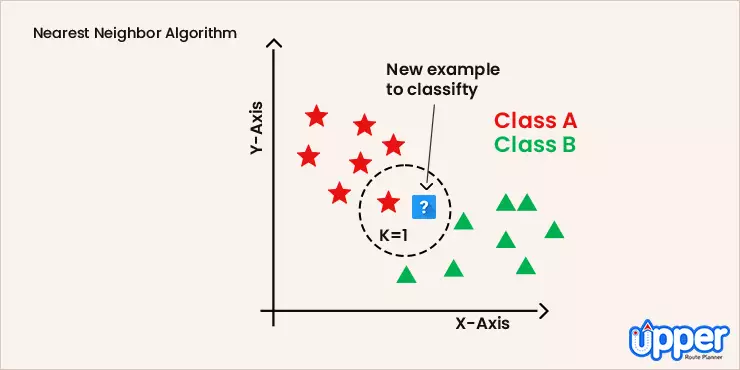The Nearest Neighbor Method is probably the most basic TSP heuristic. The method followed by this algorithm states that the driver must start by visiting the nearest destination or closest city. Once all the cities in the loop are covered, the driver can head back to the starting point.

Solving TSP using this efficient method, requires the user to choose a city at random and then move on to the closest unvisited city and so on. Once all the cities on the map are covered, you must return to the city you started from.

## 2. The Branch and Bound Algorithm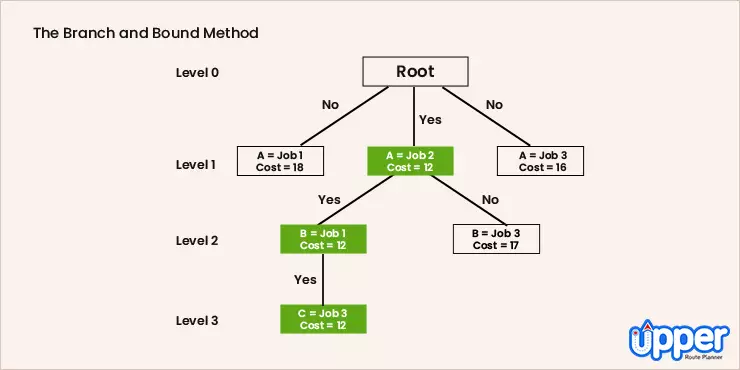The Branch & Bound method follows the technique of breaking one problem into several little chunks of problems. So it solves a series of problems. Each of these sub-problems may have multiple solutions. The solution you choose for one problem may have an effect on the solutions of subsequent sub-problems.

## 3. The Brute Force Algorithm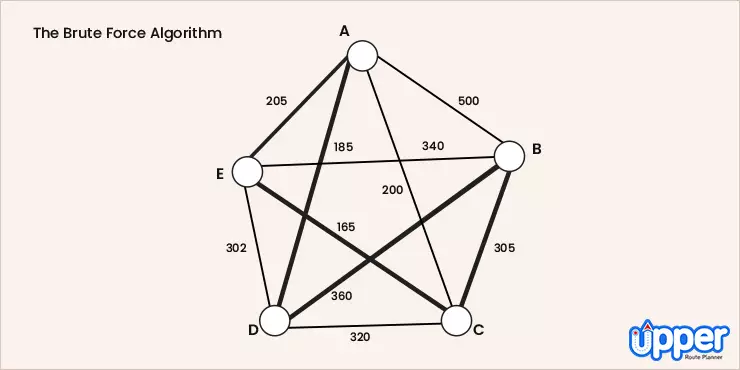The Brute Force Approach takes into consideration all possible minimum cost permutation of routes using a dynamic programming approach. First, calculate the total number of routes. Draw and list all the possible routes that you get from the calculation. The distance of each route must be calculated and the shortest route will be the most optimal solution.• Multi-Agent System : Involves distributing the pair of cities into groups. Then assign a single agent to discover the shortest path, covering all the cities in the assigned group.
• Zero Suffix Method : This method solves the classical symmetric TSP and was introduced by Indian researchers.
• Multi-Objective Evolutionary Algorithm : This method solves the TSP using NSGA-II
• Biogeography-based Optimization Algorithm : This method is based on the migration strategy of animals for solving optimization issues.
• Meta-Heuristic Multi Restart Iterated Local Search : This method states that the technique is more efficient compared to genetic algorithms.

Blow Away TSP using Upper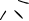Most businesses see a rise in the Traveling Salesman Problem (TSP) due to the last mile delivery challenges . The last mile delivery is the process of delivering goods from the warehouse (or a depot) to the customer’s preferred location. Considering the supply chain management, it is the last mile deliveries that cost you a wholesome amount.

At the same time, you need to sacrifice financial loss in order to maintain your current position in the market. Suppose last mile delivery costs you $11, the customer will pay$8 and you would suffer a loss. This is because of pre-defined norms which may favor the customer to pay less amount.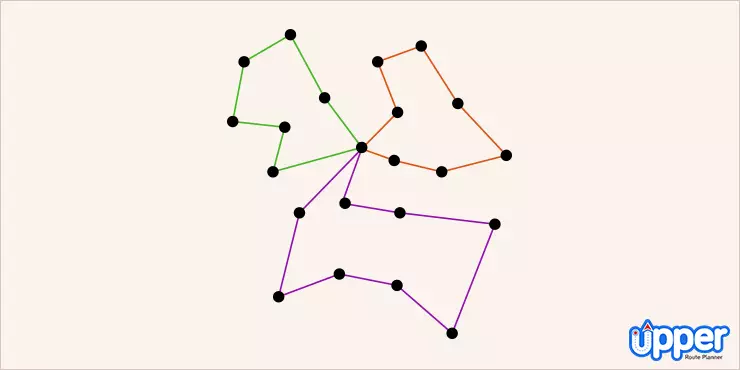This hefty last mile delivery cost is the result of a lack of Vehicle routing problem(VRP) software. VRP finds you the most efficient routes so that operational costs will not get increase. So, by using the right VRP software, you would not have to bother about TSP.

Such delivery management software uses an automated process that doesn’t need manual intervention or calculations to pick the best routes. Hence, it is the easiest way to get rid of the traveling Salesman Problem (TSP).

## How TSP and VRP Combinedly Pile Up Challenges?

The traveling Salesman Problem is an optimization problem studied in graph theory and the field of operations research. In this optimization problem, the nodes or cities on the graph are all connected using direct edges or routes. The weight of each edge indicates the distance covered on the route between the two cities.

The problem is about finding an optimal route that visits each city once and returns to the starting and ending point after covering all cities once.

The TSP is often studied in a generalized version which is the Vehicle Routing Problem . It is one of the most broadly worked on problems in mathematical optimization. VRP deals with finding or creating a set of routes for reducing time, fuel, and delivery costs.

## Is there any real world solution to TSP and VRP?

Many solutions for TSP and VRP are based on academics which means they are not so practical in everyday life. The reason is that many of them are just limited to perfection, but need a dynamic programming-based solution. So, if businesses really want to get rid of them, they need a TSP solver integrated with route optimization software.

The right TSP solver will help you disperse such modern challenges. It offers in-built route planning and optimization solutions in such a way that your tradesman doesn’t get stranded while delivering the parcel. Also, it is equipped with an efficient algorithm that provides true solutions to the TSP. As a result, the delivery manager can create a route plan hassle-free in a few minutes.

## Can a Route Planner Resolve the Traveling Salesman Problem (TSP)?

In the general case, the Traveling Salesman Problem (TSP) involves finding the shortest optimized and possible route that includes a set of stops and returns to the starting point. The number of possible routes increases exponentially as the number of locations increases. Finding the best solution becomes difficult computationally, even for moderately sized problems.

But, Upper Route Planner, a route optimization software , is built differently. Upper has all the solutions you need when talking about TSP.

For example, if you are in charge of planning delivery routes with more than 500 stops in them, all you need to do is import an Excel or CSV file with multiple addresses into Upper, review, allot delivery drivers, optimize, and dispatch with a single click. This delivery route planning solution saves you hours of time spent on planning delivery routes and optimizing them.

Also, once the delivery is completed, Upper lets you collect proof of delivery. This is how the Upper Route Planner is a simple solution to the Traveling Salesman Problem.

Upper Route Planner

A simple-to-use route planner that every one is talking about

TSP stands for traveling Salesman Problem, while VRP is an abbreviation form of vehicle routing problem (VRP). In the delivery industry, both of them are widely known by their abbreviation form.

Yes, you can prevent TSP by using the right route planner. The online route planner helps you get the optimized path so that your delivery agents don’t have to deal with such challenges. In addition, they don’t struggle with multiple routes. Instead, they can progress on the shortest route.

The new method has made it possible to find solutions that are almost as good. This was done by the Christofides algorithm, the popular algorithm in theoretical computer science. This algorithm plugs into an alternate version of the problem that finds a combination of paths as per permutations of cities. It made the round trip route much longer. The round trip produced by the new method, while still not being efficient enough is better than the old one.

The vehicle routing problem (VRP) reduces the transportation costs as well as drivers’ expenses. It helps you serve more customers with fewer fleets and drivers. Thus, you don’t have any variation in the time taken to travel.

## Create Optimized Routes Using Upper and Bid Goodbye to Traveling Salesman Problem

As a business owner, If you are dealing with TSP and want to get rid of them, we recommend using a TSP solver like Upper Route Planner. The online route planner is capable of plucking out the most efficient routes no matter how big your TSP is. It has an in-built sophisticated algorithm that helps you get the optimized path in a matter of seconds.

Therefore, you won’t fall prey to such real-world problems and perform deliveries in minimum time. Upper’s delivery route planner offers a dedicated driver app that makes sure your tradesman doesn’t go wrongfooted and quickly wraps up pending deliveries. Don’t just agree with our words, book a demo on Upper and disperse TSP once and for all.Rakesh Patel, author of two defining books on reverse geotagging, is a trusted authority in routing and logistics. His innovative solutions at Upper Route Planner have simplified logistics for businesses across the board. A thought leader in the field, Rakesh's insights are shaping the future of modern-day logistics, making him your go-to expert for all things route optimization. Read more.

Get weekly updates from Upper Route Planner.

Related PostsSales Route Planning Guide: Know How to Plan the Best Sales RoutesStress-Free Route Planning Plan. Dispatch. Track.

https://www.upperinc.com/guides/travelling-salesman-problem/

Grab a FREE Trial of Upper

• Plan routes with hundreds of stops in a minute
• Schedule routes months in advance
• Collect reliable proof of delivery
• Track drivers live for real-time updates
• Experience unparalleled customer support

Grab a FREE Trial of Upper TODAY!

• Schedule routes in advance for weeks
• Collect proof of delivery to maintain accountability
• Smart reporting to get real-time insights
• Work With Us## Three different methods to solve the travelling salesman problem

• Post author: baobab
• Post published: 1 Oct, 2020
• Post category: Algorithm## The travelling salesman problem

The travelling salesman problem (TSP) consists on finding the shortest single path that, given a list of cities and distances between them, visits all the cities only once and returns to the origin city.

Its origin is unclear. A German handbook for the travelling salesman from 1832 mentions the problem and includes example tours across Germany and Switzerland, but it does not cover its mathematics.

The first mathematical formulation was done in the 1800s by W.R. Hamilton and Thomas Kirkman. Hamilton’s icosian game was a recreational puzzle based on finding a Hamiltonian cycle, which is actually a solution to the TSP problem in a graph.

The TSP can be formulated as an integer linear program. Although several formulations are known to solve this problem, two of them are the most prominent: the one proposed by Miller, Tucker and Zemlin, and the one proposed by Dantzig, Fulkerson and Johnson.

These methods can theoretically return an optimal solution to the problem but as this is considered an NP-hard problem, they can be too expensive both in computation power and time.

In order to obtain good solutions in a shorter time, a lot of effort has been made to try and solve this problem with a variety of heuristic methods. Out of this whole group of heuristics, we would like to highlight those inspired in biology: Ant Colony Optimization (ACO, which we talked about before here ) and Genetic Algorithms (GA).

## Integer Linear programme

As we mentioned there are two main formulations for the TSP, the proposed Miller, Tucker and Zemlin (MTZ) and the Dantzig, Fulkerson and Johnson (DFJ). Although the DFJ formulation is stronger, the MTZ formulation can be useful.

## MTZ formulation

The mathematical formulation proposed by Miller, Tucker and Zemlin is as follows:Using this set, the two variables and the parameter we can then formulate the problem as:Subject to:The first and second equations enforce the type of the different variables, the third and fourth equations ensure that each node is reached and departed only once, while the last two equations enforce that only one route crosses all the nodes.

## DFJ formulationThe first three equations are the same as in the previous formulation, and the new constraint (last one) ensures that there are no sub-tours, so that the solution returned is a single tour and not the combination of smaller tours. Because this leads to an exponential number of possible constraints, in practice it is solved with delayed column generation.

In the following graph we can see the optimal solution for a 100-node problem.## Ant colony optimization

Also known as Ant Colony System (ACS) or Ant System (AS) , it is a probabilistic technique or heuristic used to solve problems that can be reduced to finding good paths in graphs. This method was initially proposed by Marco Dorigo in 1992 in his PhD thesis.

In the following animation it can be seen how this heuristic works for a problem and evolves improving the solution over time.

In the animation the red shadows represent the amount of pheromones deposited by the ants during the calculations.

## Genetic algorithm

Genetic algorithms (GA) are heuristics inspired by the evolution process of living things. Each solution is a chromosome composed by genes which represent the different values of the variables of the solution.

Starting with an initial population, we can make the solutions “evolve” over iterations. The process consists in selecting the best half of the population from which we randomly pick pairs of chromosomes (solutions) for mating. In these mating we interchange genes from both solutions to generate new solutions (children).

After the mating process we randomly apply mutations in the resulting children in order to further explore the solution space.

Finally we select the best 50 individuals from the population (the initial plus the children) and continue the process until we converge into a solution.

In the following animation we can see how the genetic algorithm evolves for the same 100-point problem.

As it can be seen in the graphs above each heuristic provides a solution to the problem, although some better than others. When we test these three methods in more datasets we can find the following results:

The performance is calculated as the deviation between the solution provided by each method and the best solution found for that instance.

In very small instances all methods reach the same solution but as the instances get bigger the heuristics start to deviate from the best solution found by the integer linear programme. Although the genetic algorithm shows a larger deviation from the optimal solution, it can still provide a solution within a shorter time than the other methods. Probably, if we were to increase the population in order to better explore the solution space we could get better results without sacrificing processing time.

ACO is the heuristic (and the one exclusively devised for this problem) that can keep with the exact model, but it takes longer to arrive at a solution.

To solve the integer linear model we need a mathematical solver , which can be either free or commercial. For this post we used a commercial solver that actually improves the performance exponentially in contrast to the free ones. This advantage is what makes the integer linear problem faster than ACO. If we were to try free software, ACO would provide better solutions in a shorter time, using an open-source language (python).

If you like solving problems like this, baobab is your place. Check out our job offers.

Check here how these algorithms to solve the Travelling Salesman Problem are developed with python.

By Guillermo González-Santander , Project Manager at baobab.

## You Might Also Like## From complex strategic decisions to simple games## Ant Colony Optimisation Algorithm

This post has one comment.

Pingback: Successfully Implementing Mathematical Optimisation: from Concept to First Results - Numens• Español – América Latina
• Português – Brasil
• Tiếng Việt

## Traveling Salesperson Problem

This section presents an example that shows how to solve the Traveling Salesperson Problem (TSP) for the locations shown on the map below.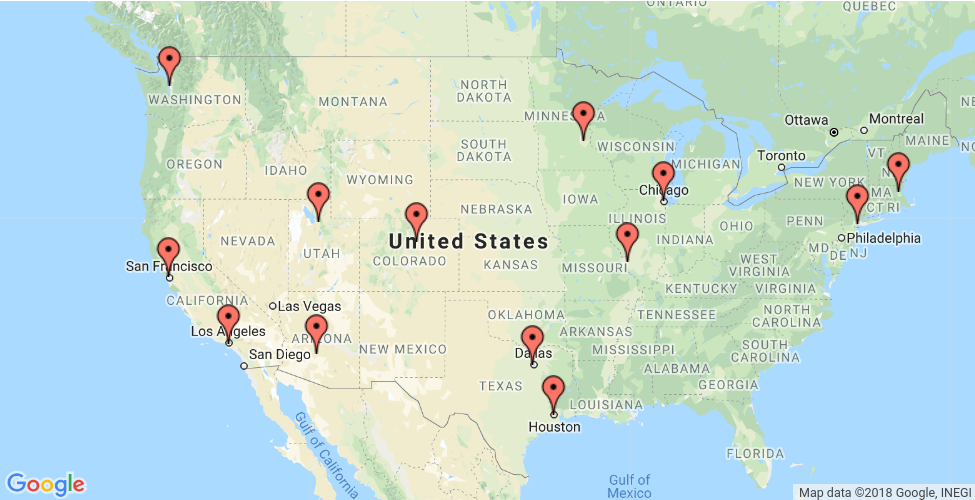The following sections present programs in Python, C++, Java, and C# that solve the TSP using OR-Tools

## Create the data

The code below creates the data for the problem.

The distance matrix is an array whose i , j entry is the distance from location i to location j in miles, where the array indices correspond to the locations in the following order:

The data also includes:

• The number of vehicles in the problem, which is 1 because this is a TSP. (For a vehicle routing problem (VRP), the number of vehicles can be greater than 1.)
• The depot : the start and end location for the route. In this case, the depot is 0, which corresponds to New York.

## Other ways to create the distance matrix

In this example, the distance matrix is explicitly defined in the program. It's also possible to use a function to calculate distances between locations: for example, the Euclidean formula for the distance between points in the plane. However, it's still more efficient to pre-compute all the distances between locations and store them in a matrix, rather than compute them at run time. See Example: drilling a circuit board for an example that creates the distance matrix this way.

Another alternative is to use the Google Maps Distance Matrix API to dynamically create a distance (or travel time) matrix for a routing problem.

## Create the routing model

The following code in the main section of the programs creates the index manager ( manager ) and the routing model ( routing ). The method manager.IndexToNode converts the solver's internal indices (which you can safely ignore) to the numbers for locations. Location numbers correspond to the indices for the distance matrix.

The inputs to RoutingIndexManager are:

• The number of rows of the distance matrix, which is the number of locations (including the depot).
• The number of vehicles in the problem.
• The node corresponding to the depot.## Create the distance callback

To use the routing solver, you need to create a distance (or transit) callback : a function that takes any pair of locations and returns the distance between them. The easiest way to do this is using the distance matrix.

The following function creates the callback and registers it with the solver as transit_callback_index .

The callback accepts two indices, from_index and to_index , and returns the corresponding entry of the distance matrix.

## Set the cost of travel

The arc cost evaluator tells the solver how to calculate the cost of travel between any two locations — in other words, the cost of the edge (or arc) joining them in the graph for the problem. The following code sets the arc cost evaluator.

In this example, the arc cost evaluator is the transit_callback_index , which is the solver's internal reference to the distance callback. This means that the cost of travel between any two locations is just the distance between them. However, in general the costs can involve other factors as well.

You can also define multiple arc cost evaluators that depend on which vehicle is traveling between locations, using the method routing.SetArcCostEvaluatorOfVehicle() . For example, if the vehicles have different speeds, you could define the cost of travel between locations to be the distance divided by the vehicle's speed — in other words, the travel time.

## Set search parameters

The following code sets the default search parameters and a heuristic method for finding the first solution:

The code sets the first solution strategy to PATH_CHEAPEST_ARC , which creates an initial route for the solver by repeatedly adding edges with the least weight that don't lead to a previously visited node (other than the depot). For other options, see First solution strategy .

The function that displays the solution returned by the solver is shown below. The function extracts the route from the solution and prints it to the console.

The function displays the optimal route and its distance, which is given by ObjectiveValue() .

## Solve and print the solution

Finally, you can call the solver and print the solution:

This returns the solution and displays the optimal route.

## Run the programs

When you run the programs, they display the following output.

In this example, there's only one route because it's a TSP. But in more general vehicle routing problems, the solution contains multiple routes.

## Save routes to a list or array

As an alternative to printing the solution directly, you can save the route (or routes, for a VRP) to a list or array. This has the advantage of making the routes available in case you want to do something with them later. For example, you could run the program several times with different parameters and save the routes in the returned solutions to a file for comparison.

The following functions save the routes in the solution to any VRP (possibly with multiple vehicles) as a list (Python) or an array (C++).

You can use these functions to get the routes in any of the VRP examples in the Routing section.

The following code displays the routes.

For the current example, this code returns the following route:

As an exercise, modify the code above to format the output the same way as the solution printer for the program.

## Complete programs

The complete TSP programs are shown below.

## Example: drilling a circuit board

The next example involves drilling holes in a circuit board with an automated drill. The problem is to find the shortest route for the drill to take on the board in order to drill all of the required holes. The example is taken from TSPLIB, a library of TSP problems.

Here's scatter chart of the locations for the holes:

The following sections present programs that find a good solution to the circuit board problem, using the solver's default search parameters. After that, we'll show how to find a better solution by changing the search strategy .

The data for the problem consist of 280 points in the plane, shown in the scatter chart above. The program creates the data in an array of ordered pairs corresponding to the points in the plane, as shown below.

## Compute the distance matrix

The function below computes the Euclidean distance between any two points in the data and stores it in an array. Because the routing solver works over the integers, the function rounds the computed distances to integers. Rounding doesn't affect the solution in this example, but might in other cases. See Scaling the distance matrix for a way to avoid possible rounding issues.

The code that creates the distance callback is almost the same as in the previous example. However, in this case the program calls the function that computes the distance matrix before adding the callback.

## Solution printer

The following function prints the solution to the console. To keep the output more compact, the function displays just the indices of the locations in the route.

## Main function

The main function is essentially the same as the one in the previous example , but also includes a call to the function that creates the distance matrix.

## Running the program

The complete programs are shown in the next section . When you run the program, it displays the following route:

Here's a graph of the corresponding route:

The OR-Tools library finds the above tour very quickly: in less than a second on a typical computer. The total length of the above tour is 2790.

Here are the complete programs for the circuit board example.

## Changing the search strategy

The routing solver does not always return the optimal solution to a TSP, because routing problems are computationally intractable. For instance, the solution returned in the previous example is not the optimal route.

To find a better solution, you can use a more advanced search strategy, called guided local search , which enables the solver to escape a local minimum — a solution that is shorter than all nearby routes, but which is not the global minimum. After moving away from the local minimum, the solver continues the search.

The examples below show how to set a guided local search for the circuit board example.

For other local search strategies, see Local search options .

The examples above also enable logging for the search. While logging isn't required, it can be useful for debugging.

When you run the program after making the changes shown above, you get the following solution, which is shorter than the solution shown in the previous section .

For more search options, see Routing Options .

The best algorithms can now routinely solve TSP instances with tens of thousands of nodes. (The record at the time of writing is the pla85900 instance in TSPLIB, a VLSI application with 85,900 nodes. For certain instances with millions of nodes, solutions have been found guaranteed to be within 1% of an optimal tour.)

## Scaling the distance matrix

Since the routing solver works over the integers, if your distance matrix has non-integer entries, you have to round the distances to integers. If some distances are small, rounding can affect the solution.

To avoid any issue with rounding, you can scale the distance matrix: multiply all entries of the matrix by a large number — say 100. This multiplies the length of any route by a factor of 100, but it doesn't change the solution. The advantage is that now when you round the matrix entries, the rounding amount (which is at most 0.5), is very small compared to the distances, so it won't affect the solution significantly.

If you scale the distance matrix, you also need to change the solution printer to divide the scaled route lengths by the scaling factor, so that it displays the unscaled distances of the routes.

Last updated 2023-01-16 UTC.

Thank you for visiting nature.com. You are using a browser version with limited support for CSS. To obtain the best experience, we recommend you use a more up to date browser (or turn off compatibility mode in Internet Explorer). In the meantime, to ensure continued support, we are displaying the site without styles and JavaScript.

• View all journals
• Explore content
• Publish with us
• Open access
• Published: 20 July 2023

## Traveling salesman problem solution using magnonic combinatorial device

• Mykhaylo Balinskyy 1 &
• Aleksandr Khitun 1

Scientific Reports volume  13 , Article number:  11708 ( 2023 ) Cite this article

856 Accesses

Metrics details

• Applied physics
• Electrical and electronic engineering
• Electronics, photonics and device physics
• Information theory and computation

Traveling salesman problem (TSP) is a decision-making problem that is essential for a number of practical applications. Today, this problem is solved on digital computers exploiting Boolean-type architecture by checking one by one a number of possible routes. In this work, we describe a special type of hardware for the TSP solution. It is a magnonic combinatorial device comprising magnetic and electric parts connected in the active ring circuit. There is a number of possible propagation routes in the magnetic mesh made of phase shifters, frequency filters, and attenuators. The phase shifters mimic cities in TSP while the distance between the cities is encoded in the signal attenuation. The set of frequency filters makes the waves on different frequencies propagate through the different routes. The principle of operation is based on the classical wave superposition. There is a number of waves coming in all possible routes in parallel accumulating different phase shifts and amplitude damping. However, only the wave(s) that accumulates the certain phase shift will be amplified by the electric part. The amplification comes first to the waves that possess the minimum propagation losses. It makes this type of device suitable for TSP solution, where waves are similar to the salesmen traveling in all possible routes at a time. We present the results of numerical modeling illustrating the TSP solutions for four and six cities. Also, we present experimental data for the TSP solution with four cities. The prototype device is built of commercially available components including magnetic phase shifters/filters, coaxial cables, splitters, attenuators, and a broadband amplifier. There are three examples of finding the shortest route between the cities for three different sets of city-to-city distances. The proposed approach is scalable to TSP with a larger number of cities. Physical limits and challenges are also discussed.

## Introduction

TSP is one of the most well-known combinatorial optimization problems 1 . It can be stated as follows: "Given a list of cities and the distances between each pair of cities, find the shortest possible route that visits each city exactly once and returns to the origin city." It is a non-deterministic polynomial-time hardness (NP-hard) problem which means that there is no guarantee to get the optimal route and no exact algorithm to solve it in polynomial time. TSP is important in a variety of practical applications including transportation 2 , scheduling 3 , and genomics 4 . Mathematically, it can be defined as given a set of $$n$$ cities, named $$\left\{{c}_{1},{c}_{2},\dots ,{c}_{n}\right\}$$ , and permutations $$\left\{{\sigma }_{1},{\sigma }_{2},\dots ,{\sigma }_{n!}\right\}$$ . The objective is to choose $${\sigma }_{i}$$ such that the sum of all Euclidean distances between cities in a tour is minimized. TSP can be modeled as an undirected weighted graph as shown in Fig.  1 , where the cities are the graph's vertices, paths are the graph's edges, and a path's distance is the edge's weight. TSP can be solved by checking one by one all possible $$\left(n-1\right)!/2$$ possible routes. It is relatively easy to check all possible routes for a small number of cities. For example, there are $$\left(4-1\right)!/2=3$$ routes for the TSP with four cities. The number of possible routes increases proportionally to $$n$$ factorial which makes it difficult to solve for a large number of cities using classical computing devices.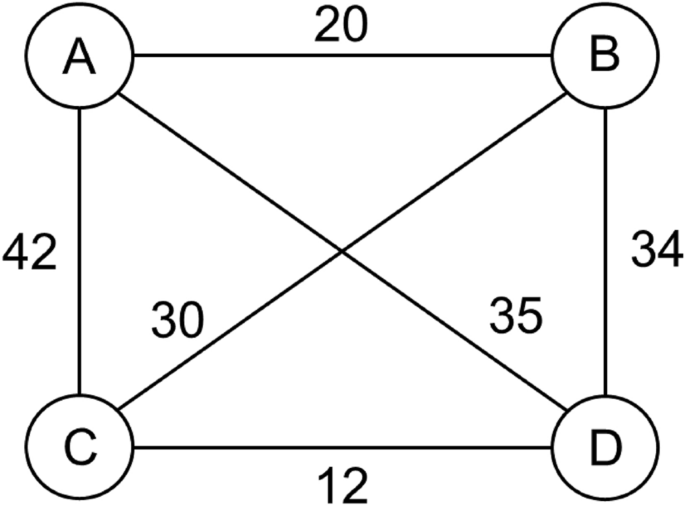Undirected weighted graph for TSP with four cities. The cities are the graph's vertices, paths are the graph's edges, and a path's distance is the edge's weight.

There were several attempts to build special hardware for TSP solution 5 , 6 . For instance, 6-city TSP was solved using a Kohonen-type optical network 6 . However, none of these approaches resulted in a practically viable device. Recently, a variety of optimization techniques used in artificial intelligence (AI) have been applied to TSP 7 . It may significantly speed up the TSP solution but cannot provide a fundamental advantage being implemented on conventional hardware.

Here, we consider a special type of combinatorial device in which an act of computation is associated with finding a propagation route of the wave through the selected nodes in the mesh. This approach was first described in Ref. 8 . The main idea is to exploit the advantage of classical wave superposition, where a number of waves can propagate through different routes at the same time. It may be possible to amplify only those signals that propagate through the selected sites in the mesh and accumulate a certain phase shift. Spin wave (magnonic) devices are most suitable for this purpose due to the prominent phase shifts that can be achieved in micrometer-length waveguides. The rest of the work is organized as follows. In “ Material structure and principle of operation ” section, we describe the principle of operation of the magnonic combinatorial logic device for TSP. The results of numerical modeling illustrating the TSP solution for four and six cities are presented in “ Numerical modeling ” section. The experimental data for the TSP solution for four cities are presented in “ Experimental data ” section. The Discussion and Conclusions are given in “ Discussion ” and “ Conclusions ” sections, respectively.

## Material structure and principle of operation

The schematics of the combinatorial device for TSP with four cities are shown in Fig.  2 . The core of the structure is the mesh consisting of the phase shifters, attenuators, frequency filters, and power sensors. The circles marked with letters A, B, C, and D, are phase shifters representing the four cities. Each phase shifter provides a unique phase shift which is proportional to the logarithm of a prime number. For example, city A is represented by the phase shifter providing $$\pi \times \mathit{Log}\left(2\right)$$ phase shift to the propagating signal. City B is represented by the phase shifter providing $$\pi \times \mathit{Log}\left(3\right)$$ phase shift, and so on. There are two cities A, one on the left and one on the right sides of the mesh. This is the city the salesman starts from and should return to after the trip. The phase shifters are connected to each other via waveguides. There is an attenuator inserted into each waveguide. The distance between the cities is encoded into the signal attenuation. For example, 10 miles can be taken equivalent to 1 dB attenuation. There is a set of frequency bandpass filters. These filters are aimed to ensure different frequencies for different propagation routes from city A on the left to city A on the right. For example, only a signal with frequency $${f}_{1}$$ can propagate through route A-B-A; only a signal with frequency $${f}_{2}$$ can propagate through route A-B-C-A, etc. There is also a set of power detectors inserted into each waveguide. These detectors are aimed to provide the output: the signal propagation route. In Fig.  2 , the detectors are marked as color circles. The green color is to depict the energy flow above some reference value (e.g., 1 dBm). The red color is to depict no energy flow.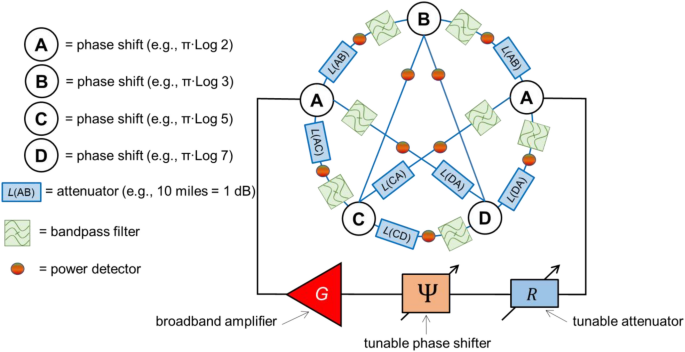Schematics of the combinatorial device for TSP with four cities. The core of the structure is the mesh consisting of the phase shifters, attenuators, frequency filters, and power sensors. The circles marked with letters A, B, C, and D, are phase shifters representing the four cities. Each phase shifter provides a unique phase shift which is proportional to the logarithm of a prime number. For example, city A is represented by the phase shifter providing $$\pi \times \mathit{Log}\left(2\right)$$ phase shift to the propagating signal. City B is represented by the phase shifter providing $$\pi \times \mathit{Log}\left(3\right)$$ phase shift, and so on. There are two cities A, one of the left and one of the right sides of the mesh. This is the city the salesman starts from and should return to after the trip. The phase shifters are connected to each via waveguides. There is an attenuator inserted between each pair of cities. The distance between the cities is encoded into the signal attenuation. For example, 10 miles can be taken equivalent to 1 dB attenuation. There is a set of frequency bandpass filters. These filters are aimed to ensure different frequencies for different propagation routes from the city A on the left to city A on the right.

There is a broadband amplifier $$G$$ , a voltage-tunable phase shifter $$\Psi$$ , and a voltage-tunable attenuator $$R$$ outside the mesh. Hereafter, we refer to these elements as the “external” part. The broadband amplifier is to provide signal amplification for all signal frequencies. The voltage-tunable phase shifter and the voltage-tunable attenuator are to control the phase shift and the attenuation of the external part. The combination of the mesh with the external part constitutes a multi-path active ring circuit 8 . There is a number of possible routes for signals to propagate in the mesh. The different propagation paths are associated with different phase shift $$\Delta ({f}_{i})$$ as well as the different signal attenuation $$L({f}_{i})$$ , where $${f}_{i}$$ is the frequency of the signal propagating through the $$i$$ -th route. There is a superposition of waves propagating through the different paths in the circuit . However, only some of the frequencies will be amplified to enable stable auto-oscillations. There are two conditions for auto-oscillations to occur in an active ring circuit 9 :

where $$G$$ is the gain provided by the amplifier, $$R$$ is the signal attenuation in the electric part, $$\Psi$$ is the phase shift of the external electric part. The first Eq. ( 1 ) states the amplitude condition for auto-oscillations: the gain provided by the broadband amplifier should be sufficient to compensate for losses in the mesh and losses introduced by the external attenuator. The second equation states the phase condition for auto-oscillations: the total phase shift for a signal circulating through the ring should be a multiple of $$2\pi .$$ In this case, signals come in phase every propagation round. A more rigorous description of signal propagation in active ring circuits can be found in Ref. 9 .

The computational procedure for TSP is the following. The external phase shifter $$\Psi$$ is set up to the value

where the last term on the right is the sum of the phase delays for the selected cities. In our case of four cities,

Only signals propagating through the selected cities (e.g., A,B,C,D, and A) will be amplified as the total phase shift through the ring is $$2\pi$$ . Signals that propagate through the selected cities but more than ones (e.g., A-C-B-D-C-A) will not be amplified because the total phase shift is not a multiple of $$2\pi$$ . A more detailed explanation of the selective signal amplification in a multi-path active ring circuit can be found in Ref. 8 . All other signal propagating on other paths (e.g., A-B-A, A-C-D-A, etc.) won’t be amplified as the phase condition ( 2 ) is not satisfied.

Then, it is possible to find the shortest path (i.e., the path with minimum losses) using the external attenuator $$R$$ . There may be a number of routes for which the total phase shift satisfies Eq. ( 2 ). The number of routes satisfying the amplitude condition Eq. ( 1 ) decreases with the increase of the external damping $$R$$ . The shortest path will disappear the last as it may sustain the maximum external damping. There are two important points we want to emphasize regarding the principle of operation. T he system starts with a superposition of all possible frequencies propagating through all possible paths. We can amplify only the signals coming through the selected nodes/cities using the external phase shifter to satisfy the phase condition ( 2 ). The shortest route is found then by introducing additional damping so only the signal with minimum losses can satisfy the amplitude condition ( 1 ). In the next section, we present the results of numerical modeling illustrating the TSP solution.

## Numerical modeling

To illustrate the solution procedure described above, we present the results of numerical modeling for the four-cities TSP. In Fig.  3 A, there is shown a map of the 20 most fun cities in U.S. (source WalletHub). The Traveling Salesman starts from Los Angeles and has to visit Miami, Washington, Chicago, and come back to Los Angeles. The distances between the cities are taken from the Google Map application. The problem is solved using the combinatorial device shown in Fig.  2 . The distances between the cities are converted into signal attenuation (e.g., 1000 miles = 10 dB attenuation).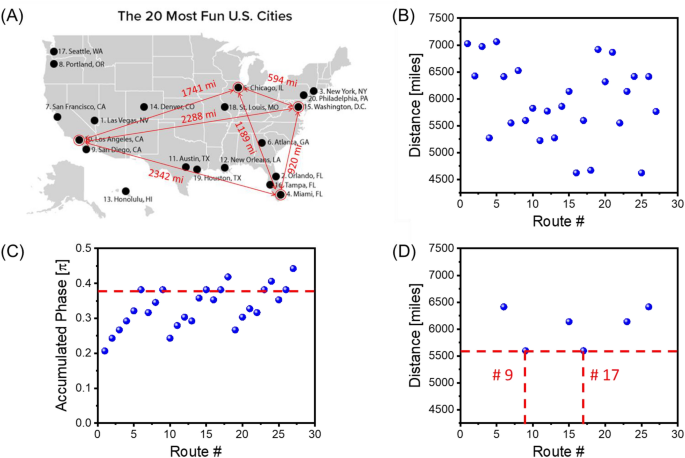( A ) U.S. map with the 20 most fun cities. The Traveling Salesman starts from Los Angeles and has to visit Miami, Washington, Chicago and come back to Los Angeles. ( B ) Results of numerical modeling showing the distances for all possible routes. The routes are marked by #1, #2, … #27. Each of the routes is associated with a continuous sinusoidal signal of some frequency $${f}_{1}$$ , $${f}_{2},\dots {f}_{27}$$ . ( C ) Results of numerical modeling showing phase shifts for all possible routes. Only 6 routes out of 27 satisfy the phase condition ( 2 ) and will be amplified. ( D ) Results of numerical modeling depicting the shortest route(s): Los Angeles–Miami–Washington–Chicago–Los Angeles and Los Angeles–Chicago–Washington–Miami–Los Angeles.

There are 27 possible routes between the four cities (e.g., Los Angles–Washington–Los Angeles–Miami–Washington–Los Angeles). In Fig.  3 B, there are shown the travel distances for all routes. The routes are marked by #1, #2, … #27. Each of the routes is associated with a continuous sinusoidal signal of some frequency $${f}_{1}$$ , $${f}_{2},\dots {f}_{27}$$ . The values of these frequencies are of no importance. We assume that the phase shifts provided by the cities do not depend on the signal frequency. The problem is solved in two steps. In Step 1, we set up the external phase shifter according to Eqs. ( 3 ) and ( 4 ). Only the routes coming through all four cities and coming back to Los Angeles will be amplified in the active ring circuit. In Fig.  3 C, there are shown phase shifts for all possible routes. Only 6 routes out of 27 satisfy the phase condition ( 2 ) and will be amplified. In Step 2, we introduce additional damping for the external electric circuit so only the route with minimum losses can satisfy condition ( 2 ). In Fig.  3 D, there are shown the results of numerical modeling depicting the shortest route(s) out of six possible from Step 1. Actually, there are always two routes with the shortest distance. In our case, these are Los Angeles–Miami–Washington–Chicago–Los Angeles and Los Angeles–Chicago –Washington–Miami–Los Angeles.

The above-described algorithm can be extended to a larger number of cities. For example, there are shown the schematics of a device for TSP with six cities in Fig.  4 . There are two more phase shifters marked as E and F added to the mesh. These shifters provide $$\pi \times \mathit{Log}\left(11\right)$$ and $$\pi \times \mathit{Log}\left(13\right)$$ phase shifts, respectively. There are more interconnects between the sites of the mesh. Each interconnect is a waveguide that includes an attenuator, a frequency filter, and a power detector. For simplicity, attenuators, filters, and detectors are not shown in Fig.  4 . In this example, the traveling salesperson starting from Los Angeles should visit Las Vegas, Chicago, Washington, Miami, San-Francisco, and return to Los Angeles. The map with the cities is shown in Fig.  5 A. The table with city-to-city distances can be found in the Supplementary materials . There are more than 3000 possible routes as shown in Fig.  5 B. Not all of these routes are coming through all the selected cities. In Step 1, the external phase shifter is tuned toSchematics of the combinatorial device for TSP with six cities. There are two more phase shifters compared to Fig.  2 marked as E and F added to the mesh. These shifters provide $$\pi \times \mathit{Log}\left(11\right)$$ and $$\pi \times \mathit{Log}\left(13\right)$$ phase shifts, respectively.( A ) U.S. map with the 20 most fun cities. The traveling salesperson starting from Los Angeles should visit Las Vegas, Chicago, Washington, Miami, San Francisco, and return to Los Angeles. ( B ) Results of numerical modeling showing the distances for all possible routes. ( C ) Results of numerical modeling showing phase shifts for all possible routes. ( D ) Results of numerical modeling depicting the shortest route(s): Los Angeles–Miami–Washington–Chicago–Las Vegas–San Francisco–Los Angeles, and the backward route: Los Angeles–San Francisco–Las Vegas–Chicago–Washington–Miami–Los Angeles.

Only routes coming through all cities (i.e., the routes accumulating the required phase shift) will be amplified. The results of numerical modeling in Fig.  5 C show the routes satisfying Eq. ( 1 ). In Step 2, additional damping is introduced by the external attenuator. In Fig.  5 D, there are shown the shortest routes (i.e., #320 and #3062) that correspond to Los Angeles–Miami–Washington–Chicago–Las Vegas–San Francisco–Los Angeles, and the route with the backward city order: Los Angeles–San Francisco–Las Vegas–Chicago–Washington–Miami–Los Angeles.

It should be noted that in the presented above examples TSP was solved using a general type computer. All possible routes were calculated one by one. Routes with a phase shift satisfying Eq. ( 1 ) were selected then. The shortest route out of the routes with the right phase was found by checking all the routes with the right phase. The use of conventional hardware does not provide any advantage over the existing algorithms. To speed up the TSP solution, one needs a device that utilized wave superposition and checks all possible routes in parallel.

## Experimental data

In this part, we present experimental data showing TSP solution for four cities. There is a variety of possible approaches to the practical implementation of the device shown in Fig.  2 . It may be a superposition of mechanical, acoustic, or electromagnetic waves propagating through all possible routes in the mesh. Our approach is aimed to explore the unique combination of properties inherent in spin waves, where magnetic waveguides can serve as phase shifters and frequency filters at the same time. Spin wave is a collective oscillation of spins in a spin lattice around the direction of magnetization. Spin waves appear in magnetically ordered structures, and a quantum of spin wave is called a “magnon” 10 . Active ring circuits with spin wave delay lines are widely used as tunable microwave sources 11 . Radiofrequency spin waves propagate in magnetic waveguides much slower compared to electromagnetic waves in coaxial cables. For instance, the group velocity of magnetostatic spin waves in a single-crystal yttrium iron garnet Y 3 Fe 2 (FeO 4 ) 3 (YIG) film of thickness 7 µm is about 10 4  m/s 12 . It makes it possible to obtain large (e.g., up to 2pi) phase shifts for the propagating signal in millimeter-length waveguides. At the same time, spin wave dispersion strongly depends on waveguide magnetization. Spin waves propagating along the direction of magnetization (so-called backward volume magnetostatic spin waves (BVMSW) and spin waves propagating perpendicular to the direction of magnetization (so-called magnetostatic surface spin waves MSSW) possess different dispersion 13 . Thus, both the phase shift and the frequency window for spin wave propagation can be controlled by the locally applied magnetic field. It may be just a micro-scale magnet placed on the top of the YIG waveguide. The first proof-of-the-concept experiment on signal re-direction in the two-path combinatorial logic device was accomplished using a YIG-based active ring circuit with BVMSW and MSSW 8 .

In this work, the mesh is built using commercially available YIG-based frequency filters produced by Micro Lambda Wireless, Inc, model MLFD-40540. The experimental data on the filter transmission and phase delay can be found in the supplementary materials . The schematics of the device are shown in Fig.  6 . In Fig.  6 A, there is shown the general view of the device similar to the one in Fig.  2 . The main difference is in the number of routes connecting the A city on the left and the A city on the right. There are shown nine band-pass filters. The central frequencies of the filters are setup to transmit three selected frequencies: $${f}_{1}=2.441$$ GHz, $${f}_{2}=2.514$$ GHz, and $${f}_{3}=2.595$$ GHz. The filters are assembled to provide three propagation routes: $${f}_{1}$$ for A-B-C-D-A, $${f}_{2}$$ for A-C-D-B-A, and $${f}_{3}$$ for A-D-B-C-A. The device is aimed to find the shortest route depending on the set of attenuators. In Fig.  6 B, there are shown the connection schematics of the prototype device. There are six double-channel bandpass frequency filters connected via coaxial cables. The two letters (e.g., AB, CD, etc.) depict the path at which the filter is introduced. For example, AB means that the filter is introduced to the path connecting cities A and B. Each of the filters introduces a phase shift to the propagating signal. This fact is exploited to minimize the number of components in the circuit The phase delay for each city is introduced by the frequency filters. The system was calibrated so the signals propagating through the three routes accumulate the same phase shifts. The amplification in the electric part is provided by three amplifiers (Mini-Circuits, model ZX60-83LN-S+) connected in series. The total amplification is about 15 dB in all experiments. There is external phase shifter (ARRA, model 9418A) which adjusted to provide the phase shift of $$\pi /6$$ . The phase condition ( 2 ) is satisfied for all three routes. Experimental data on signal propagation can be found in the supplementary materials . There are 12 attenuators in the circuit, where the attenuation in dB is proportional to the distance between the cities. The power flow is detected using a unidirectional coupler (KRYTAR, model 1850) which is attached to the 12 selected places as shown in Fig.  6 B. These places are marked with numbers 1,2,3 and 4. The marks have different colors (i.e., red, green, and blue) to separate signals propagating on frequencies $${f}_{1}$$ , $${f}_{2},$$ and $${f}_{3}$$ , respectively. The power detected at port 1 corresponds to the input power. The power measured at ports 2,3, and 4 corresponds to the signal after traveling the first, the second, and the third cities, respectively. Also, the frequency spectra of the auto-oscillation in the active ring circuit can be measured using the Spectrum Analyzer and PNA as shown in Fig.  6 B. The spectra can be found in the Supplementary materials .( A ) General view of the prototype device for TSP with four cities. There are 12 single-frequency bandpass filters included in the scheme. The central frequencies of the filters are setup to transmit three selected frequencies: $${f}_{1}=2.441$$ GHz, $${f}_{2}=2.514$$ GHz, and $${f}_{3}=2.595$$ GHz. The filters are assembled to provide three propagation routes: $${f}_{1}$$ for A-B-C-D-A, $${f}_{2}$$ for A-C-D-B-A, and $${f}_{3}$$ for A-D-B-C-A. ( B ) Connection schematics of the prototype device. The phase delay for each city is introduced by the frequency filters. The system was calibrated so the signals propagating through the three routes accumulate the same phase shifts. There are 12 attenuators in the circuit, where the attenuation in dB is proportional to the distance between the cities. The power flow is detected using a unidirectional coupler (model) which is attached to the 12 selected places marked with numbers 1,2,3, and 4. The marks have different colors (i.e., red, green, and blue) to separate signals propagating on frequencies $${f}_{1}$$ , $${f}_{2},$$ and $${f}_{3}$$ , respectively.

In the next three examples, we present experimental data demonstrating the shortest route finding depending on the set of attenuators for different paths. It should be noted that not the bandpass frequencies nor connectivity nor the position of the external phase shifter are changed during the experiments.

Experiment 1: In Fig.  7 A, there are shown the values of attenuators for different paths. These values are randomly chosen based on the available components. As soon as the magnetic and electric parts are connected, the device starts to search for the resonant path. It takes less than 100 $$\mu s$$ for coming to the stable regime of auto-oscillations. In Fig.  7 B, there are shown experimental data on the power flow in the circuit. There is about the same input power for all three possible routes. Most of the power flows through the route A-C-D-B-A. There is more than a 30 dBm difference with the other two routes (i.e., A-B-C-D-A and A-D-B-C-A), which are not in resonance with the electric part. The propagation route is illustrated in Fig.  7 C. All measurements are done at room temperature.( A ) Node-to-node attenuation. ( B ) Experimental data on the power flow in the mesh. ( C ) The shortest route ACDBA is depicted by the power sensors.

Experiment 2: Next, the set of attenuators was changed. In Fig.  8 A there are shown the values of attenuators for different paths. In Fig.  8 B, there are shown experimental data on the power flow in the circuit. There is about the same input power for all three possible routes. Most of the power flows through the route A-D-B-C-A. As in the previous example, there is more than a 30 dBm difference with the other two routes (i.e., A-B-C-D-A and A-C-D-B-A). The propagation route is illustrated in Fig.  8 C.( A ) Node-to-node attenuation for Example 2. ( B ) Experimental data on the power flow in the mesh. ( C ) The shortest route ADBCA is depicted by the power sensors.

Experiment 3: In Fig.  9 A, there is shown a different set of values of attenuation for different paths. In Fig.  9 B, there are shown experimental data on the power flow in the circuit. Most of the power flows through the route A-B-C-D-A. As in the previous example, there is more than a 30 dBm difference with the other two routes (i.e., A-B-C-D-A and A-C-D-B-A). The propagation route is illustrated in Fig.  9 C. There is an infinite number of sets for attenuators mimicking the traveling distance between the four cities. In all cases, the device will find the shortest route through all the cities.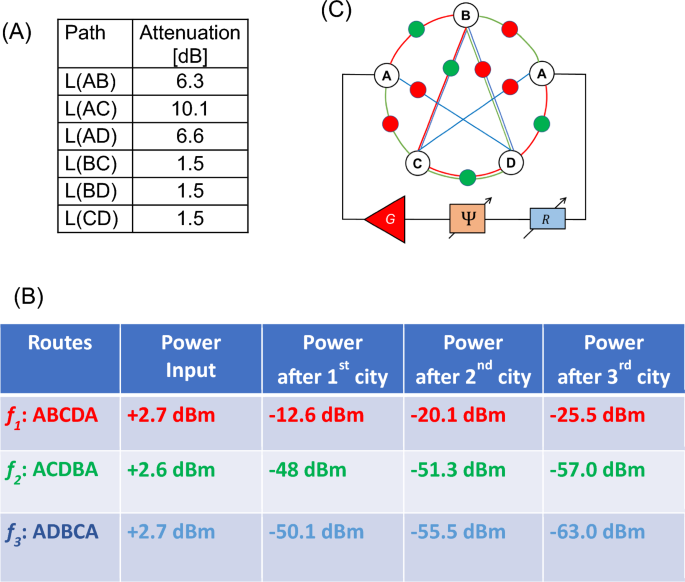( A ) Node-to-node attenuation for Example 2. ( B ) Experimental data on the power flow in the mesh. ( C ) The shortest route ABCDA is depicted by the power sensors.

There are several observations we would like to make based on the obtained experimental data. The prototype magnonic combinatorial device does show the shortest route of propagation through the selected sites in the mesh made of frequency filters, phase shifters, and attenuators. It is critically important that the change in the signal propagation route demonstrated in the examples is only due to the change in the path attenuation. Thus, the device can be utilized for all possible combinations of path attenuation (distances) between the nodes (cities).

There is a prominent difference in the power flow through the different routes which exceeds 30 dBm at room temperature. The active ring circuit device selectively amplifies only the routes which satisfy resonant conditions ( 1 ) and ( 2 ). The phase condition ( 2 ) allows us to extract the routes with desired accumulated phase change. The amplitude condition ( 1 ) makes it possible to select only the shortest route out of many with the same accumulated phase. It takes a special step in numerical modeling for extracting the shortest route by increasing the external attenuation $$R$$ . The extraction of the shortest route happens naturally in the prototype device due to the non-linear phenomena. This effect is known as mode suppression 14 , 15 which is observed in mechanical, acoustic, and optical resonators. It explains the energy re-distribution of power between the modes, where the mode with minimum attenuation receives the most of the amplification. It is one rare case when the experimental prototype works more efficiently than the theoretical device for numerical modeling.

It should be noted the difference between the general-type device shown in Fig.  2 and the prototype shown in Fig.  4 . It is assumed that all routes connecting the A city on the left and the A city on the right are available in the general-type device. As mentioned before, there are “parasitic” routes like A-B-A, A-C-B-A which do not go through all the cities. The “right” routes are selected using the external phase shifter. The device shown in Fig.  2 can be used for finding any route (e.g., A-B-A, A-C-D-A) by adjusting the external phase shifter. In contrast, there are only three routes (i.e., A-B-C-D-A, A-B-D-C-A, and A-C-B-D-A) in the device shown in Fig.  4 . It is also possible to include “parasitic” routes in the cost of additional frequency filters. For example, one would need to include two additional frequency filters tuned to the central frequency $${f}_{4}$$ to have route A-B-A.

There is a variety of approaches to practical implementation of combinatorial devices. Magnonic approach has certain advantages (e.g., prominent phase shift for RF signal in micro-meter scale waveguides) and disadvantages (e.g., prominent spin wave damping). It may be possible to build optical combinatorial devices with low damping and fast signal propagation if the prominent phase delay can be achieved. The ability to exploit classical wave superposition provides a fundamental advantage over conventional digital computers in functional throughput. Functional throughput is a commonly accepted metric for logic devices evaluation 16 , which can be defined as follows:

Conventional logic devices based on Complementary Metal-Oxide Semiconductor (CMOS) technology possess deep sub-micrometer characteristic size (e.g., 7 nm channel length) and perform one operation in a very short time (e.g., a fraction of nanosecond) 17 . However, these Boolean-type devices can accomplish only one operation at a time. In contrast, combinatorial logic devices are efficient in parallel search. This search through multiple routes is equivalent to the number of subsequent operations for the digital computer. The number of possible routes in TSP scales proportional to $$\left(n-1\right)!/2.$$ The time of computation scales proportionally to $$n\cdot l/{v}_{g},$$ where $$l$$ is the length of the waveguide connecting the nodes in the mesh, $${v}_{g}$$ is the group velocity of the signal. The area of the mesh scales proportionally to $$\sqrt{n}\cdot {l}^{2}$$ . The functional throughput of the combinatorial devices for TSP can be estimated as follows:

Taking the length of the waveguide to be 10 mm and the group velocity to be $${10}^{4}$$ m/s, the functional throughput is skyrocketing to $${10}^{35}$$ Ops/s∙m 2 which is above the limits of the existing supercomputers combined.

Surprisingly, there is a lot of similarity in solving different problems such as the Bridges of Konigsberg, TSP, and prime factorization. The use of prime numbers for marking unique mesh nodes (i.e., cities in TSP) looks very efficient and guarantees the uniqueness of the phase accumulated through the different routes. In turn, the same device can be used for prime factorization. The external phase shifter can be set to $$\Psi =2\pi -\pi Log(X)$$ , where $$X$$ is the number to be factorized. The device will find a route at which the accumulated phase matches the external phase. The examples of prime factorization with the combinatorial logic device are presented in the preceding work 8 . In the future, it may be possible to build a universal combinatorial device capable of solving a variety of NP-hard and NP problems.

The key practical question is related to the number of parts (i.e., waveguides, frequency filters, attenuators, power detectors) for building a device for TSP with a large number of cities. In Table 1 , there are shown the estimates for the number of parts for TSP with $$n$$ cities. The number of phase shifters for cities scales as $$n+1$$ . One extra phase shifter is needed for the second A city. The number of waveguides connecting the phase shifters is given by

where the first term on the right accounts for the number of paths in the classical TSP as shown in Fig.  2 . The second term on the right accounts for the additional $$\left(n-1\right)$$ waveguides for the second city A. There is the same number of attenuators and power detectors required for TSP with $$n$$ cities. A more complicated is situation with frequency filters. There are 12 single-frequency bandpass filters (4 filters for 3 routes) shown in Fig.  6 A. In the prototype, we used separate cables and frequency filters to make two propagation paths between some cities (e.g., BC and DC). It would take an enormous amount $$\left(n-1\right)!$$ of single-frequency bandpass filters for TSP with $$n$$ cities following this approach. In general, an $$i-th$$ route in the combinatorial device should be associated with some frequency $${f}_{i}$$ . It leads to the grand challenge of the presented approach as the number of possible routes increases factorial with the number of cities. The number of distinct frequencies can be quite large (e.g., 1 M frequencies in the frequency range from 0.5 to 10 GHz with the inter-frequency separation of 0.5 MHz). However, it is sufficient for solving TSP with just 6–7 cities. In part, this problem can be resolved by utilizing the same frequency for not-crossing routes. The problem can be resolved completely by utilizing combinatorial filters transmitting signals comprising waves with a certain combination of frequencies (e.g., $${\{f}_{1}$$ , $${f}_{2},$$ $${f}_{5}$$ , $${f}_{11}\}$$ or $${\{f}_{2}$$ , $${f}_{4},$$ $${f}_{7}$$ , $${f}_{19}\},$$ etc.). In this scenario, the number of filters is the same as the number of waveguides given by Eq. ( 8 ), where each filter has a unique combination of the bandpass frequencies. In all cases, there is only one external broadband amplifier, one external tunable phase shifter, and one external tunable attenuator.

It should be noted that every new problem requires circuit adjustment or reconfiguration. For instance, the circuit for four cities presented in this work can be used for any other TSP with four cities. One needs to adjust the attenuation according to the distances between the cities. It can be done using voltage-tunable attenuators. The increase in the number of cities requires an increase in the number of phase shifters in the circuit. However, TSP circuits can be used for a smaller number of cities without removing/adding new components. For example, TSP with eight cities can be used for solving problems for eight, seven, six, five, and four cities.

Another practical challenge is associated with the accuracy of the parts with respect to the number of cities. The difference in the distances between the cities may significantly decrease for TSPs with a large number of cities. There are physical limits to the accuracy of attenuator control. Also, the increase of possible propagation routes inevitably decreases the difference in the accumulated phase for different routes. In turn, it will lead to the problem with the shortest route finding for a large number of cities. The increase in the precision of measurements/calculations is a common problem for TSP with a large number of cities that may be addressed with heuristic algorithms 18 . Less accuracy is required for the power detectors. A prominent difference in the power flow (e.g., a 20 dB difference between the active and passive routes) can be achieved regardless of the number of cities. There are certain pros and cons of using magnonic circuits for TSP. On the one hand, spin waves provide prominent phase shifts on millimeter-length waveguides. On the other hand, spin wave dispersion significantly complicates circuit engineering. Besides, spin waves possess much stronger interaction compared to optical waves. For instance, spin waves propagating in the orthogonal directions through the junction may completely reflect each other 19 . It constitutes an additional problem for spin wave interconnects. This and many other questions deserve special consideration.

## Conclusions

We described an approach to TSP solution using combinatorial devices. The principle of operation is based on the classical wave superposition, where each wave corresponds to a traveling salesman. The waves propagate through a mesh of waveguides, where phase shifters represent the cities in TSP and the distance between the cities is encoded in the signal attenuation. Only waves coming through the selected cities and accumulating the specific phase shift are amplified. The most amplified is the wave traveling through the route with minimum attenuation. TSP solution is illustrated by numerical modeling and experimentally demonstrated. The first prototype is a multi-path magnonic active ring circuit comprising magnetic phase shifters, frequency filters, and attenuators. The signal propagation route is detected via the power detector. We presented thee examples for three different sets of attenuators/city-to-city distances. The device literally finds the shortest propagating route through the cities. The operation is robust as the power difference between the active and passive routes exceeds 30 dBm. All experiments are accomplished at room temperature. This work is a step toward combinatorial logic devices for special task data processing. Potentially, combinatorial devices may provide a fundamental advantage in functional throughput compared to conventional digital devices. The ability to use classical superposition of waves is the key advantage and the most appealing property of the presented approach.

## Data availability

All data generated or analyzed during this study are included in this published article.

Biggs, N., Lloyd, E. K. & Wilson, R. J. Graph theory, 1736–1936. Isis 70 , 164–165. https://doi.org/10.1086/352170 (1979).

Ungureanu, V. Traveling salesman problem with transportation. Comput. Sci. J. Mold. 14 , 202–206 (2006).

Fuentes, G. E. A., Gress, E. S. H., Mora, J. & Marin, J. M. Solution of the job-shop scheduling problem through the traveling salesman problem. Rev. Iberoam. Autom. Inform. Ind. 13 , 430–437. https://doi.org/10.1016/j.riai.2016.07.003 (2016).

Gusfield, D. & Gusfield, D. Traveling Salesman Problems in Genomics (Cambridge Univ Press, 2019).

Aarts, E. H. L. & Korst, J. H. M. Boltzmann machines for traveling salesman problems. Eur. J. Oper. Res. 39 , 79–95. https://doi.org/10.1016/0377-2217(89)90355-x (1989).

Collings, N., Sumi, R., Weible, K. J., Acklin, B. & Xue, W. In International Topical Meeting on Optical Computing (Oc 92). 637–641 (Spie-Int Soc Optical Engineering, 1993).

Saud, S., Kodaz, H. & Babaoglu, I. In 9th International Conference on Advances in Information Technology (IAIT). 17–32 (Knowledge E, 2018).

Khitun, A. & Balinskiy, M. Combinatorial logic devices based on a multi-path active ring circuit. Sci. Rep. https://doi.org/10.1038/s41598-022-13614-2 (2022).

Article   PubMed   PubMed Central   Google Scholar

Tiberkevich, V. S., Khymyn, R. S., Tang, H. X. & Slavin, A. N. Sensitivity to external signals and synchronization properties of a non-isochronous auto-oscillator with delayed feedback. Sci. Rep. https://doi.org/10.1038/srep03873 (2014).

Kittel, C. Introduction to Solid State Physics 8th edn. (Wiley, 2005).

Chumak, A. V., Serga, A. A. & Hillebrands, B. Magnonic crystals for data processing. J. Phys. D-Appl. Phys. 50 , 1–20. https://doi.org/10.1088/1361-6463/aa6a65 (2017).

Balinskiy, M. et al. Spin wave interference in YIG cross junction. Aip Adv. https://doi.org/10.1063/1.4974526 (2017).

Gurevich, A. G. & Melkov, G. A. Magnetization Oscillations and Waves 147–176 (CRC Press, 1996).

Okusaga, O. et al. In 2010 IEEE International Frequency Control Symposium. 539–543 (IEEE, 2010).

Carroll, J. M. & Chang, K. Microstrip mode suppression ring-resonator. Electron. Lett. 30 , 1861–1862. https://doi.org/10.1049/el:19941291 (1994).

Nikonov, D. E. & Young, I. A. Uniform methodology for benchmarking beyond-CMOS logic devices. In 2012 IEEE International Electron Devices Meeting (IEDM 2012) , vol. 25, https://doi.org/10.1109/iedm.2012.6479102 (2012).

Hoefflinger, B. In Chips 2020, Vol. 2: New Vistas in Nanoelectronics Frontiers Collection (ed, Hoefflinger, B.) 143–148 (Springer, 2016).

Syambas, N. R., Salsabila, S., Suranegara, G. M. & IEEE. In 11th International Conference on Telecommunication Systems Services and Applications (TSSA). (IEEE, 2017).

Balynskiy, M. et al. Reversible magnetic logic gates based on spin wave interference. J. Appl. Phys. https://doi.org/10.1063/1.5011772 (2018).

## Acknowledgements

This work of M. Balinskiy and A. Khitun was supported in part by the INTEL CORPORATION, under Award #008635, Project director Dr. D. E. Nikonov, and by the National Science Foundation (NSF) under Award # 2006290, Program Officer Dr. S. Basu. The authors would like to thank Dr. D. E. Nikonov for the valuable discussions.

## Author information

Authors and affiliations.

Department of Electrical and Computer Engineering, University of California-Riverside, Riverside, CA, 92521, USA

Mykhaylo Balinskyy & Aleksandr Khitun

You can also search for this author in PubMed   Google Scholar

## Contributions

A.K. conceived the idea of combinatorial magnonic devices for TSP and wrote the manuscript, M.B. built the prototype and carried out the experiments. All authors discussed the data and contributed to the manuscript preparation.

## Corresponding author

Correspondence to Aleksandr Khitun .

## Ethics declarations

Competing interests.

The authors declare no competing interests.

Publisher's note.

Springer Nature remains neutral with regard to jurisdictional claims in published maps and institutional affiliations.

## Supplementary Information

Supplementary information., rights and permissions.

Reprints and Permissions

Balinskyy, M., Khitun, A. Traveling salesman problem solution using magnonic combinatorial device. Sci Rep 13 , 11708 (2023). https://doi.org/10.1038/s41598-023-38839-7

Accepted : 16 July 2023

Published : 20 July 2023

DOI : https://doi.org/10.1038/s41598-023-38839-7

Anyone you share the following link with will be able to read this content:

Provided by the Springer Nature SharedIt content-sharing initiative

By submitting a comment you agree to abide by our Terms and Community Guidelines . If you find something abusive or that does not comply with our terms or guidelines please flag it as inappropriate.

• Explore articles by subject
• Guide to authors
• Editorial policies## Computer Scientists Break Traveling Salesperson Record

October 8, 2020Islenia Mil for Quanta Magazine

## Introduction

When Nathan Klein started graduate school two years ago, his advisers proposed a modest plan: to work together on one of the most famous, long-standing problems in theoretical computer science.

Even if they didn’t manage to solve it, they figured, Klein would learn a lot in the process. He went along with the idea. “I didn’t know to be intimidated,” he said. “I was just a first-year grad student — I don’t know what’s going on.”

Now, in a paper posted online in July, Klein and his advisers at the University of Washington, Anna Karlin and Shayan Oveis Gharan , have finally achieved a goal computer scientists have pursued for nearly half a century: a better way to find approximate solutions to the traveling salesperson problem.

This optimization problem, which seeks the shortest (or least expensive) round trip through a collection of cities, has applications ranging from DNA sequencing to ride-sharing logistics. Over the decades, it has inspired many of the most fundamental advances in computer science, helping to illuminate the power of techniques such as linear programming. But researchers have yet to fully explore its possibilities — and not for want of trying.

The traveling salesperson problem “isn’t a problem, it’s an addiction,” as  Christos Papadimitriou , a leading expert in computational complexity, is fond of saying.

Most computer scientists believe that there is no algorithm that can efficiently find the best solutions for all possible combinations of cities. But in 1976, Nicos Christofides came up with an algorithm that efficiently finds approximate solutions — round trips that are at most 50% longer than the best round trip. At the time, computer scientists expected that someone would soon improve on Christofides’ simple algorithm and come closer to the true solution. But the anticipated progress did not arrive.

“A lot of people spent countless hours trying to improve this result,” said Amin Saberi of Stanford University.

Now Karlin, Klein and Oveis Gharan have proved that an algorithm devised a decade ago beats Christofides’ 50% factor, though they were only able to subtract 0.2 billionth of a trillionth of a trillionth of a percent. Yet this minuscule improvement breaks through both a theoretical logjam and a psychological one. Researchers hope that it will open the floodgates to further improvements.Nathan Klein (left), a graduate student at the University of Washington, and his advisers, Anna Karlin and Shayan Oveis Gharan.

Flora Hollifield; from “ Embracing Frustration ,” with permission from Microsoft; courtesy of Shayan Gharan.

“This is a result I have wanted all my career,” said David Williamson of Cornell University, who has been studying the traveling salesperson problem since the 1980s.

The traveling salesperson problem is one of a handful of foundational problems that theoretical computer scientists turn to again and again to test the limits of efficient computation. The new result “is the first step towards showing that the frontiers of efficient computation are in fact better than what we thought,” Williamson said.

## Fractional Progress

While there is probably no efficient method that always finds the shortest trip, it is possible to find something almost as good: the shortest tree connecting all the cities, meaning a network of connections (or “edges”) with no closed loops. Christofides’ algorithm uses this tree as the backbone for a round-trip tour, adding extra edges to convert it into a round trip.

Any round-trip route must have an even number of edges into each city, since every arrival is followed by a departure. It turns out that the reverse is also true — if every city in a network has an even number of connections then the edges of the network must trace a round trip.

The shortest tree connecting all the cities lacks this evenness property, since any city at the end of a branch has just one connection to another city. So to turn the shortest tree into a round trip, Christofides (who died last year) found the best way to connect pairs of cities that have odd numbers of edges. Then he proved that the resulting round trip will never be more than 50% longer than the best possible round trip.

In doing so, he devised perhaps the most famous approximation algorithm in theoretical computer science — one that usually forms the first example in textbooks and courses.

“Everybody knows the simple algorithm,” said Alantha Newman of Grenoble Alpes University and the National Center for Scientific Research in France. And when you know it, she said, “you know the state of the art” — at least, you did until this past July.

Computer scientists have long suspected that there should be an approximation algorithm that outperforms Christofides’ algorithm. After all, his simple and intuitive algorithm isn’t always such an effective way to design a traveling salesperson route, since the shortest tree connecting the cities may not be the best backbone you could choose. For instance, if this tree has many branches, each city at the end of a branch will need to be matched with another city, potentially forming lots of expensive new connections.

In 2010, Oveis Gharan, Saberi and Mohit Singh of the Georgia Institute of Technology started wondering if it might be possible to improve on Christofides’ algorithm by choosing not the shortest tree connecting all the cities, but a random tree from a carefully chosen collection. They took inspiration from an alternate version of the traveling salesperson problem in which you are allowed to travel along a combination of paths — maybe you get to Denver via 3/4 of the route from Chicago to Denver plus 1/4 of the route from Los Angeles to Denver.

Unlike the regular traveling salesperson problem, this fractional problem can be solved efficiently. And while fractional routes don’t make physical sense, computer scientists have long believed that the best fractional route should be a rough guide to the contours of good ordinary routes.

So to create their algorithm, Oveis Gharan, Saberi and Singh defined a random process that picks a tree connecting all the cities, so that the probability that a given edge is in the tree equals that edge’s fraction in the best fractional route. There are many such random processes, so the researchers chose one that tends to produce trees with many evenly connected cities. After this random process spits out a specific tree, their algorithm plugs it into Christofides’ scheme for matching cities with odd numbers of edges, to convert it into a round trip.

This method seemed promising, not just to the three researchers but to other computer scientists. “The intuition is simple,” said Ola Svensson of the Swiss Federal Institute of Technology Lausanne. But “to prove it turns out to be a different beast.”

The following year, though, Oveis Gharan, Saberi and Singh managed to prove that their algorithm beats Christofides’ algorithm for “graphical” traveling salesperson problems — ones where the distances between cities are represented by a network (not necessarily including all connections) in which every edge has the same length. But the researchers couldn’t figure out how to extend their result to the general traveling salesperson problem, in which some edges may be vastly longer than others.

“If we have to add a super expensive edge to the matching then we’re screwed, basically,” Karlin said.

## Pushing Back

Nevertheless, Oveis Gharan emerged from that collaboration with an unshakable belief that their algorithm should beat Christofides’ algorithm for the general traveling salesperson problem. “I never had a doubt,” he said.

Oveis Gharan kept turning the problem over in his mind over the years that followed. He suspected that a mathematical discipline called the geometry of polynomials, little known in the theoretical computer science world, might have the tools he needed. So when Karlin came to him two years ago suggesting that they co-advise a brilliant new graduate student named Nathan Klein who had double-majored in math and cello, he said, “OK, let’s give it a try — I have this interesting problem.”

Karlin thought that, if nothing else, it would be a fun opportunity to learn more about the geometry of polynomials. “I really didn’t think we would be able to solve this problem,” she said.

She and Oveis Gharan had no hesitation about throwing Klein into the deep end of computer science research. Oveis Gharan had himself cut his teeth on the traveling salesperson problem as a graduate student back in 2010. And the two advisers agreed about the merits of assigning hard problems to graduate students, especially during their first couple of years, when they are not under pressure to get results.

The three dived into an intense collaboration. “It’s all I was thinking about for two years,” Klein said.

They spent the first year solving a simplified version of the problem, to get a sense of the challenges they were facing. But even after they accomplished that, the general case still felt like a “moonshot,” Klein said.

Still, they had gotten a feel for their tools — in particular, the geometry of polynomials. A polynomial is a combination of terms made out of numbers and variables raised to powers, such as 3 x 2 y + 8 xz 7 . To study the traveling salesperson problem, the researchers distilled a map of cities down to a polynomial that had one variable for each edge between cities, and one term for each tree that could connect all the cities. Numerical factors then weighted these terms to reflect each edge’s value in the fractional solution to the traveling salesperson problem.

This polynomial, they found, has a coveted property called “real stability,” which means that the complex numbers that make the polynomial evaluate to zero never lie in the upper half of the complex plane. The nice thing about real stability is that it stays in force even when you make many kinds of changes to your polynomial. So, for example, if the researchers wanted to focus on particular cities, they could use a single variable to represent all the different edges leading into a city, and they could set the variables for edges they didn’t care about equal to 1. As they manipulated these simplified polynomials, the results of their manipulations still had real stability, opening the door to a wide assortment of techniques.

This approach enabled the researchers to get a handle on questions like how often the algorithm would be forced to connect two distant cities. In a nearly 80-page analysis, they managed to show that the algorithm beats out Christofides’ algorithm for the general traveling salesperson problem (the paper has yet to be peer-reviewed, but experts are confident that it’s correct). Once the paper was completed, Oveis Gharan dashed off an email to Saberi, his old doctoral adviser. “I guess I can finally graduate,” he joked.Amin Saberi (left) of Stanford University and Mohit Singh of the Georgia Institute of Technology.

Courtesy of Amin Saberi; Lance Davies

While the improvement the researchers established is vanishingly small, computer scientists hope this breakthrough will inspire rapid further progress. That’s what happened back in 2011 when Oveis Gharan, Saberi and Singh figured out the graphical case. Within a year, other researchers had come up with radically different algorithms that greatly improved the approximation factor for the graphical case, which has now been lowered to 40% instead of Christofides’ 50%.

“When they announced their result [about the graphical case], … that made us think that it’s possible. It made us work for it,” said Svensson, one of the researchers who made further progress in that case. He’s been trying for many years to beat Christofides’ algorithm for the general traveling salesperson problem. “I will try again now I know it’s possible,” he said.

Over the decades, the traveling salesperson problem has launched many new methods into prominence. Oveis Gharan hopes that it will now play that role for the geometry of polynomials, for which he has become an eager evangelist. In the decade or so since he started learning about this approach, it has helped him prove a wide range of theorems. The tool has “shaped my whole career,” he said.

The new traveling salesperson result highlights the power of this approach, Newman said. “Definitely it’s an inspiration to look at it more closely.”

Klein will now have to find a new problem to obsess over. “It’s a bit sad to lose the problem, because it just built up so many structures in my head, and now they’re all kind of gone,” he said. But he couldn’t have asked for a more satisfying introduction to computer science research. “I felt like we pushed back a little bit on something that was unknown.”

This article was reprinted on Wired.com and in Italian at  le Scienze .

Get highlights of the most important news delivered to your email inbox

Quanta Magazine moderates comments to facilitate an informed, substantive, civil conversation. Abusive, profane, self-promotional, misleading, incoherent or off-topic comments will be rejected. Moderators are staffed during regular business hours (New York time) and can only accept comments written in English.## Next article#### IMAGES

1. The Travelling Salesman Problem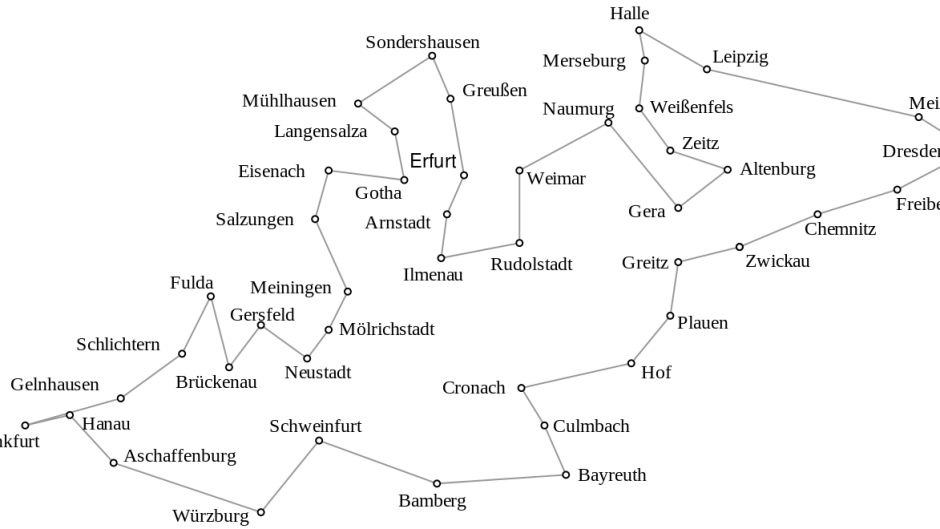2. PPT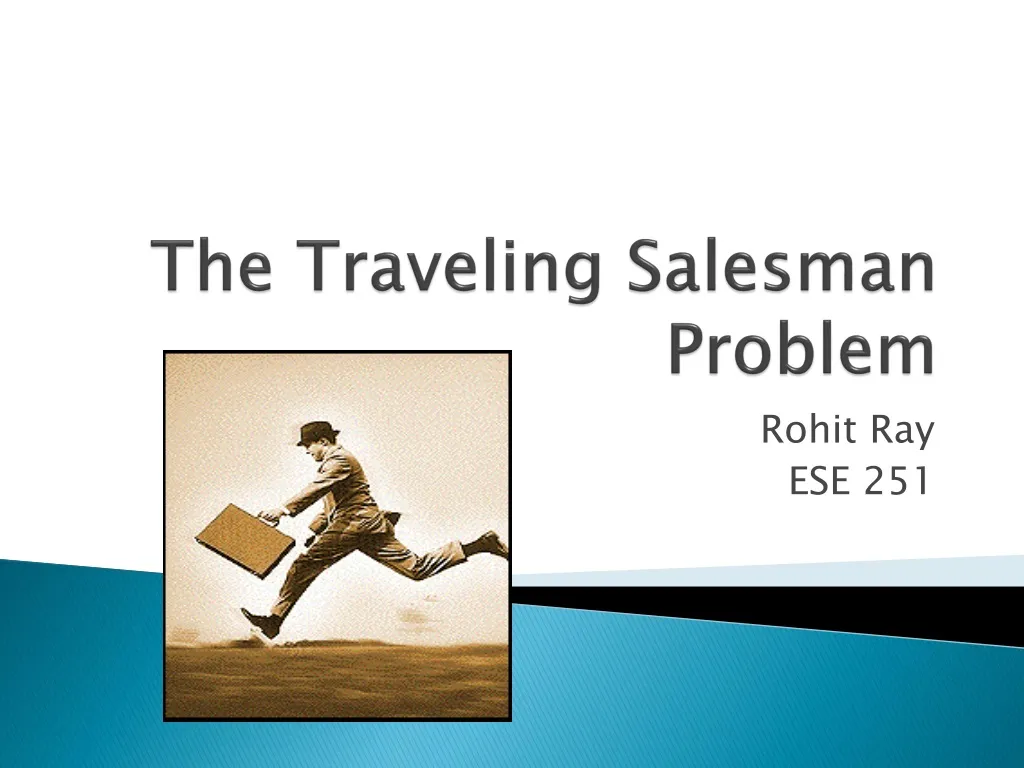3. PPT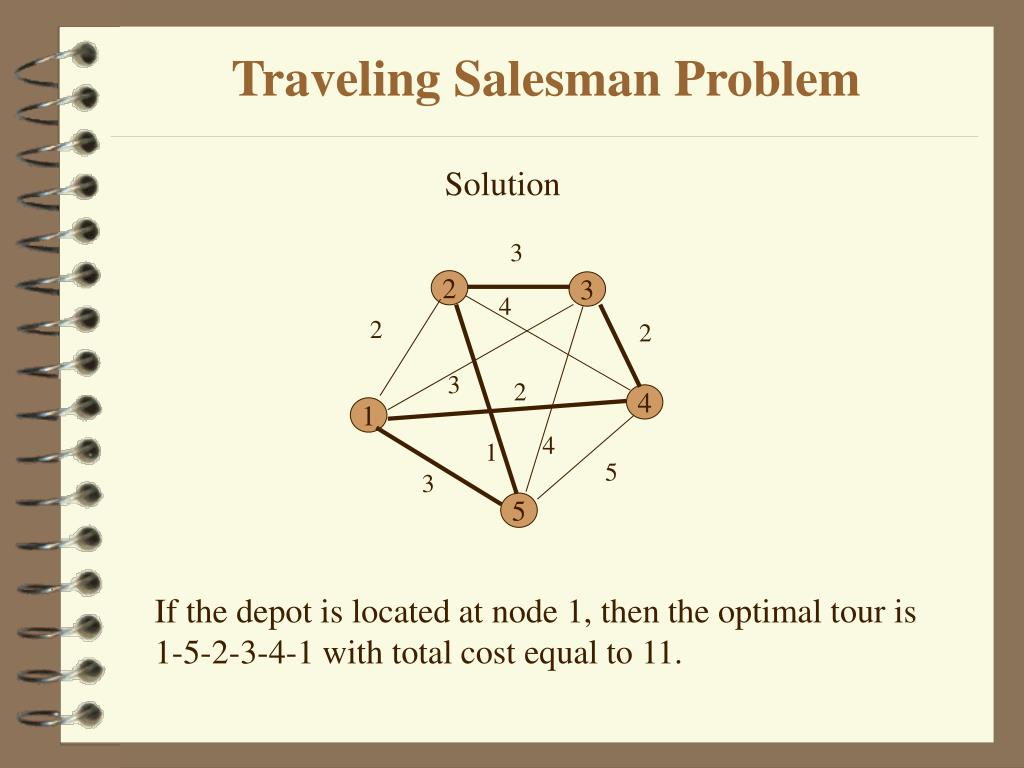4. The Traveling Salesman Problem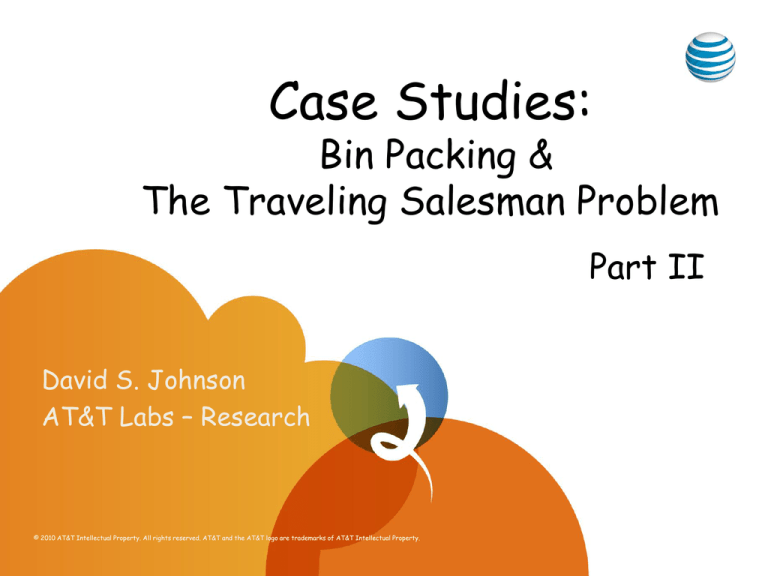5. Travelling Salesman Problem (TSP) using Different Approaches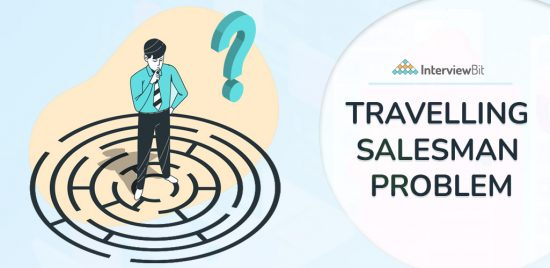6. PPT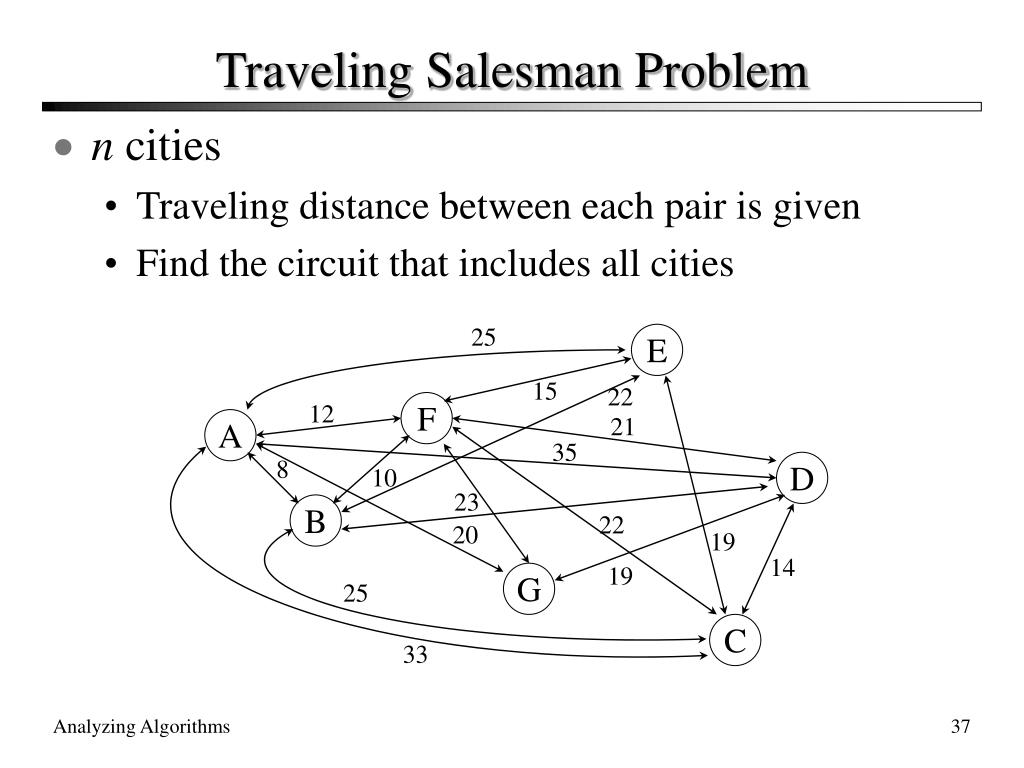#### VIDEO

1. Is the Traveling Salesman Problem (TSP)an NP-Complete Problem?

2. Travelling Salesman Problem

3. Traveling Salesman Problem [Greedy + VNS]

4. Sabancı Üniversitesi

5. Leetcode Weekly contest 359

6. Travelling Salesman Problem using Dynamic Programming || Hindi || By Studies Studio

1. What Are Some Solutions to Environmental Problems?

A variety of solutions for environmental problems exist including recycling, reduction of carbon emissions from fossil fuels, finding alternative energy solutions and the conservation of marine life.

2. What Are Example Statistics and Probability Problems and Their Solutions?

Two examples of probability and statistics problems include finding the probability of outcomes from a single dice roll and the mean of outcomes from a series of dice rolls. The most-basic example of a simple probability problem is the clas...

3. What Are Some Solutions to Social Problems?

Sexually transmitted infections and unwanted pregnancies can be partly solved by comprehensive sex education. Job creation and work support are some solutions to hunger and poverty. Social support and access to health care are some solution...

4. Algorithms for the Travelling Salesman Problem

To solve the TSP using the Brute-Force approach, you must calculate the total number of routes and then draw and list all the possible routes.

5. Travelling salesman problem

The Beardwood–Halton–Hammersley theorem provides a practical solution to the travelling salesman problem. The authors derived an asymptotic formula to determine

6. Travelling Salesman Problem using Dynamic Programming

Travelling Salesman Problem (TSP):. Given a set of cities and the distance between every pair of cities, the problem is to find the shortest

7. Traveling Salesman Problem (TSP) Implementation

Traveling Salesman Problem (TSP) Implementation · Consider city 1 as the starting and ending point. Since the route is cyclic, we can consider

8. Explained: What is Traveling Salesman Problem (TSP)

What are Some Popular Solutions to Traveling Salesman Problem? · 1. Nearest Neighbor (NN) · 2. The Branch and Bound Algorithm · 3. The Brute Force

9. Three different methods to solve the travelling salesman problem

The first and second equations enforce the type of the different variables, the third and fourth equations ensure that each node is reached and

10. Traveling Salesperson Problem

Create the data · Create the routing model · Create the distance callback · Set the cost of travel · Set search parameters · Add the solution printer · Solve and

11. Traveling salesman problem solution using magnonic combinatorial

Traveling salesman problem (TSP) is a decision-making problem that is essential for a number of practical applications. Today, this problem

12. How to Solve the Traveling Salesman Problem

The traveling salesman problem is a classic problem in combinatorial optimization. This problem is to find the shortest path that a salesman

13. Computer Scientists Break Traveling Salesperson Record

The Travelling Salesman Problem finds the shortest route between all the nodes, but doesn't have to use all the edges, because the sales

14. The Traveling Salesman Problem 14 different solutions

The Traveling Salesman Problem (TSP) is a classic optimization problem in computer science and mathematics. It involves finding the shortest